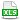HomeTechniques and Tips@RISK Distribution FittingRMS Error Calculation in Distribution Fitting with (x,p) Pairs

# 4.6. RMS Error Calculation in Distribution Fitting with (x,p) Pairs

Applies to: @RISK 5.x–7.x

How does @RISK calculate the RMS error that it uses for fit ranking?

For curve data—x values with associated probability densities or cumulative probabilities—@RISK computes the root-mean-square error as a measure of goodness of fit. The equation is in the help file, but it can be hard to relate that to the computations for your particular data set.

The attached example shows how @RISK computes the RMS error for (x,p) data, where p is the cumulative probability or area under the curve for all values less than or equal to that x value.

Last edited: 2015-06-19

•RMSError_Example.xls